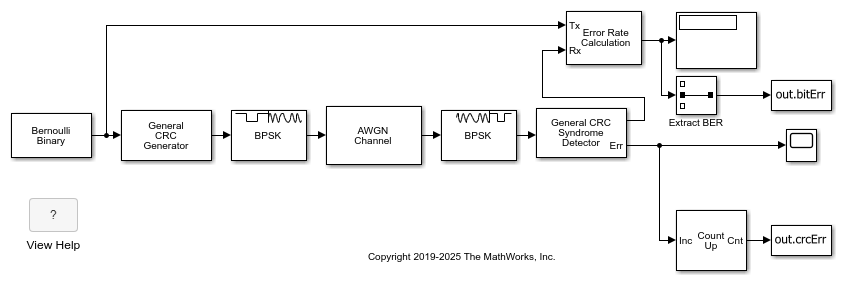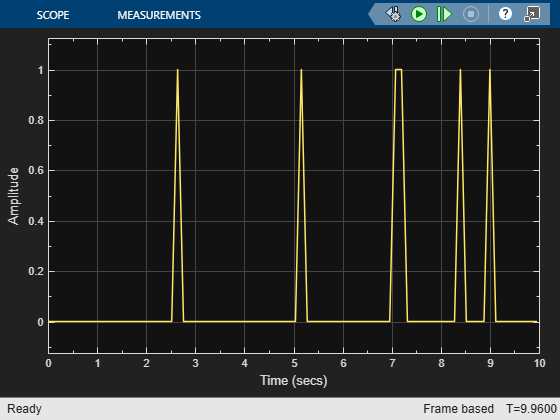# Cyclic Redundancy Check of Noisy BPSK Data Frames in Simulink

Use a CRC code to detect frame errors in a noisy BPSK signal.

The CRC generator and detector pair use a standard CRC-4 polynomial,. The length of the CRC is 4 bits as determined my the degree of the polynomial. The number of checksums per frame is 1, so the full transmission frame has one CRC appended at the end.

A binary signal frame gets a CRC code appended to the end of the frame. BSPK modulation is applied to the signal and the signal passes through an AWGN channel. The signal is demodulated, and then a CRC syndrome detector removes the CRC and calculates the CRC errors.Generate 12-bit frames of binary data and append CRC bits. Based on the degree of the polynomial, 4 bits are appended to each frame. Apply BPSK modulation and pass the signal through an AWGN channel. Demodulate and use the CRC detector to determine if the frame is in error.The results of the CRC detection are compared to a BER calculation.

```Number of bit errors detected: 6 Number of crc errors detected: 7 ```